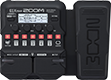# Bir Derdim Var Solo

Discussion in 'Zoom G1/G1X Four' started by KIRRIK, Jan 31, 2022.

1. Bir Derdim Var SoloDevice: Zoom G1X Four
Firmware: 2.00

Name on device: Cln Delay
Optimized for: Phones/Speaker

Effects chain:Ibanez GRG170DXL

Effect: "ZNR" (Dynamics), active - "yes"
"Detect" = GTRIN
"Depth" = 100
"Threshold" = 52
"Decay" = 0

Effect: "FD B-MAN" (Amp simulator), active - "yes"
"Input" = BRIGHT
"Bass" = 38
"Middle" = 107
"Treble" = 79
"Presence" = 83
"Gain" = 30
"Volume" = 120

Effect: "FD-B4x10" (Cabinet), active - "yes"
"MIC" = ON
"D57: D421" = 0
"Hi" = 55
"Lo" = 50

Effect: "St Gt GEQ" (Filter), active - "yes"
"160" = 0.0
"400" = 0.0
"800" = 0.0
"3.2k" = 0.0
"6.4k" = 0.0
"12k" = 0.0
"VOL" = 80

Effect: "Delay" (Delay), active - "yes"
"Time" = 353
"F.B." = 17
"Mix" = 38
"Tail" = Off

Patch Volume: 90